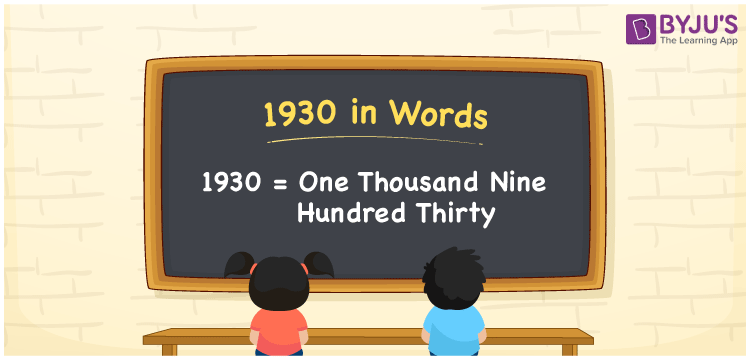# 1930 in Words

One Thousand Nine Hundred Thirty is the numerical name of the number 1930. For instance, Rashmi purchased a table fan worth Rs. 1930, then you can say, “Rashmi purchased a table fan worth Rupees One Thousand Nine Hundred Thirty”. 1930 is a cardinal number. We can convert the number 1930 into words quickly and easily using a place value system. Let us have a look at how to convert the number 1930 in word form with the help of a place value chart and the interesting facts about 1930 in a descriptive manner.

 1930 in Words One Thousand Nine Hundred Thirty One Thousand Nine Hundred Thirty in numerical form 1930

## 1930 in English Words

Usually, we write numbers in words using the English alphabet. Therefore, we can write and read the number 1930 in English as One Thousand Nine Hundred Thirty.## How to Write 1930 in Words?

Find the place value of each digit in the number 1930 before converting it into words. We need a place value chart of 4 columns as 1930 is a four-digit number. The following table depicts the place value chart for the number 1930.

 Thousands Hundreds Tens Ones 1 9 3 0

Therefore, we can write the expanded form as:

1 x Thousand + 9 x Hundred + 3 x Ten + 0 x One

= 1 x 1000 + 9 x 100 + 3 x 10 + 0 x 1

= 1000 + 900 + 30 + 0

= 1000 + 900 + 30

= 1930

= One Thousand Nine Hundred Thirty

Hence, 1930 in words is written as One Thousand Nine Hundred Thirty

Interesting way of writing 1930 in words

1 = One

19 = Nineteen

193 = One Hundred and Ninety-Three

1930 = One Thousand Nine Hundred Thirty

Thus, the word form of the number 1930 is One Thousand Nine Hundred Thirty

1930 is a natural number that is the successor of 1929 and the predecessor of 1931

• 1930 in words – One Thousand Nine Hundred Thirty
• Is 1930 an odd number? – No
• Is 1930 an even number? – Yes
• Is 1930 a perfect square number? – No
• Is 1930 a perfect cube number? – No
• Is 1930 a prime number? – No
• Is 1930 a composite number? – Yes

## Frequently Asked Questions on 19300 in Words

Q1

### Write 1930 in words.

1930 in words is written as One Thousand Nine Hundred Thirty.
Q2

### Simplify 1000 + 930, and express in words.

Simplifying 1000 + 930, we get 1930. Thus, 1930 in words is One Thousand Nine Hundred Thirty.
Q3

### 1930 is an odd number. True or False.

False, the number 1930 is not an odd number.